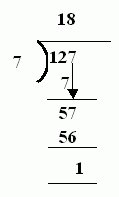Name: ___________________Date:___________________

 Email us to get an instant 20% discount on highly effective K-12 Math & English kwizNET Programs!

### MEAP Preparation - Grade 4 Mathematics1.3 Division With Remainders

 Look at the larger number which is the dividend. The lesser number is called divisor. The dividend is divided by the divisor. If the divisor is not exactly divisible by the divisor, it results in a remainder. Example: 127/718 times 7 gives 126. Answer: 18R1 Directions: Divide the following. Also write at least 10 examples of your own.Name: ___________________Date:___________________

### MEAP Preparation - Grade 4 Mathematics1.3 Division With Remainders

 Q 1: Which is the correct answer to 75/8?9R39R18R4none of these Q 2: Which is the correct answer to 14/3?2R13R2none of these4R2 Q 3: Which is the correct answer to 84/9?9R3none of these7R68R2 Q 4: Which is the correct answer to 27/5?none of these5R14R35R2 Q 5: Which is the correct answer to 46/7?none of these5R46R46R3 Q 6: Which is the correct answer to 55/98R1none of these6R15R4 Q 7: Which is the correct answer to 18/4?6R35R4none of these4R2 Q 8: Which is the correct answer to 66/8?none of these8R17R58R2 Question 9: This question is available to subscribers only! Question 10: This question is available to subscribers only!